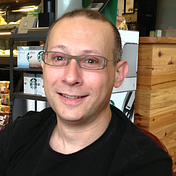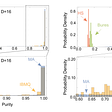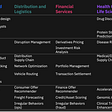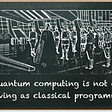# Demystifying Superdense Coding

In this article I will help to demystify the magic behind quantum superdense coding along with some sample code that you can run yourself on IBM’s Quantum Experience so you can see it in action. We will be using QISKit so download and install the SDK.

In a nutshell superdense coding enables us to send two classical bits of information by only manipulating a single quantum bit known simply as a qubit.

I will walk you through the math, quantum circuits, and code. Along the way, I will explain what is happening in each step. Before I get started I assume that you have some knowledge of qubits, Dirac notation, classical bits, and linear algebra. If you are looking for a tutorial on Quantum Computing I encourage you to check out the IBM Q Experience documentation.

## Introduction

To start our journey into understanding superdense coding, we need to first discuss two fundamental quantum computing concepts: superposition and entanglement. I will only provide a brief overview of these concepts. If you want a more detailed explanation, then take a look at this excellent tutorial from Benoit Valîron.

Before we dive into the foundational concepts let’s look at some Python code that we will use in our examples. In the following code fragment, we create two types of registers: quantum and classical. The classical registers will be used to store the results of measuring our quantum registers. The quantum registers are our ‘qubits’ and we will manipulate them in our program to achieve certain desired effects. The quantum circuit will be used to wire our qubits and operations together. You can find a copy of the code that we will use throughout the article here.

`from qiskit import QuantumCircuit, ClassicalRegister, QuantumRegisterfrom qiskit import available_backends, execute# Create two quantum and classical registersq = QuantumRegister(2)c = ClassicalRegister(2)qc = QuantumCircuit(q, c)`

## Superposition

Quantum superposition is a special qubit probabilistic state where there is an equal chance of obtaining the binary value 0 or 1 when the qubit is measured. This state is constructed by applying a special operation to a qubit called the Hadamard operator. The Hadamard operator is a unitary transformation that causes a qubit that is either |0⟩ or |1⟩ in the standard basis to be transformed into a new state that is called superposition.

As a refresher recall the Dirac and vector notation for |0⟩ and|1⟩ and the general description of a qubit as a linear combination of basis states, as shown in the diagrams below.

In the diagrams below the matrix corresponding to the Hadamard operator is applied to a qubit in the |0⟩ basis state. The resultant vector has equal probabilities of returning either 0 or 1 when the qubit is measured.

The probabilities are calculated by taking the sum of the squares of the absolute values of the magnitudes. In this case, we obtain a 50% chance of measuring either 0 or 1.

In quantum circuit diagrams the Hadamard operator is often referred to as the Hadamard gate and is represented by an H enclosed in a box. The diagram below is the quantum circuit that corresponds to the previous matrix operations.

Here we see the Hadamard operator being applied to a qubit in the |0⟩ basis followed by a measurement to obtain the classical bit value 0 or 1. The code fragment shows how to apply the Hadamard operator to one of our qubits.

`# Add a Hadamard gate on qubit 0 to create a superpositionqc.h(q)`

## Entanglement and Bell States

Quantum entanglement is a special state of strong correlation between two qubits even when the qubits are physically separated from each other by great distances. The correlation is so strong that the qubits appear to be communicating faster than the speed of light.

Measuring one of the qubits instantaneously determines the value of the other even when the other qubit is in a probabilistic state. This idea is often the most difficult to comprehend as it is completely at odds with our everyday experiences and may take some time getting used to.

As a point of reference, Albert Einstein was never comfortable with the idea of non-deterministic measurements and entanglement, referring to this as “spooky action at a distance”. Einstein argued that Quantum Mechanics must be incomplete, because we know that no information can be communicated faster than the speed of light. There must be some hidden variable that we are unable to detect that explains the strong correlation and the appearance of faster than light communication.

In 1964 John Bell devised an experiment to test whether there was a hidden variable being used that could explain the strong correlation. I will not go into the details of the experiment, but Bell proved that there was no such hidden variable being shared between two entangled qubits and the qubits are not communicating faster than the speed of light. If you want to learn more you can take a look at the details here. The important takeaway from this experiment is that there are four unique distinguishable orthogonal entangled states as shown below that are often called the Bell states (Φ+,Φ−,Ψ+,Ψ−).

What do these Bell states mean? These Bell states tell us that there is an equal probability of a pair of entangled qubits either being in the first configuration or the second configuration in any one of the given four Bell states. For example, in the first Bell state Φ+ the qubits have an equal chance of being either in the |00⟩ or |11⟩ configuration, while in the third Bell state Ψ+ the qubits have an equal chance of being either in the |01⟩ or |10⟩ configuration.

The notion of entanglement that is often difficult to comprehend is that the entangled state cannot be decomposed into separate states from each of the two qubits. The diagram below illustrates how we end up with a contradiction when we try to take the product of two qubits in an attempt to generate the Φ+ state and therfore our entangled state is some new “mixed state” that is not describable by the individual qubit states.

At this point you are probably asking yourself how do two qubits become entangled and how is this useful? Before I dive into answering these questions I need to introduce another quantum operator called the controlled not operator or CNOT.

The CNOT operator is a 2 qubit unitary transformation where one qubit acts as a control and the other serves as a target. If the control qubit is set to 1, then the target qubit is flipped. The matrix shown below describes this operator.

In a quantum circuit diagram the CNOT gate appears as a black dot on the quantum wire for the control qubit with a line and circle connecting it to the target’s qubit quantum wire, as shown in the diagram below.

So now back to the first of our earlier questions that being, how do we create a pair of entangled qubits? Simply put we are going to combine the use of the Hadamard gate with the CNOT gate. The following quantum circuit creates an entangled pair of qubits in one of the four Bell states that we listed earlier, as shown below. The Bell state that is produced is contingent upon the initial states of the qubits.

The diagram below shows the effect of combining the Hadamard operator and the CNOT gate to generate the first Bell state Φ+ given both qubits start in the |0⟩ initial state. If we wanted to generate any of the other Bell states, then we would simply change the initial states of the qubits. The code fragment below shows us adding the CNOT gate to our qubits, remember that qubit 0 is already in superposition from the earlier step.

`# Add a controlled not (CX) with qubit 0 as control and qubit 1 as target# This causes the two qubits to become entangled qc.cx(q, q)`

So back to our second question of how will this be useful? Given that we can vary the Bell states that get generated based upon the initial qubit conditions we can use that as a foundation for communicating information.

Let’s assume for the moment that we wanted to recover the initial conditions of the qubits prior to entanglement how would be able to do that? The answer to that is very straightforward. We simply need to mirror the use of the controlled not and Hadamard gates, as shown in the diagram below. By doing this we can undo the effects of the prior Hadamard and CNOT gates that put the qubits into an entangled state. This is possible, because quantum gate operators are unitary transformations that are reversible. Reapplying these operators returns us to the original initial qubit conditions.

This means that if we were to give someone a pair of entangled qubits in some unknown Bell state all they would have to do to recover the initial conditions is to simply apply the CNOT and Hadamard gates to the pair of qubits. Once this is performed all that is left to do is to measure the qubits which reveals the initial qubit states. In the next section, we are going to extend this idea a little bit to cleverly communicate two classical bits of information by only manipulating one of the entangled qubits.

## Superdense Coding

Now we are armed with nearly all the information that we need to understand superdense coding. The only remaining details that we need to discuss are two quantum operators (Pauli X and Pauli Z) that are collectively known as Pauli operators or gates, as shown in the diagram below. These gates are single qubit gates and as we will they see simply rotate our qubit vector. With these operators, we can explain how the superdense coding algorithm works.

In most literature on this subject the concept of superdense coding is described as two colleagues (Alice and Bob) wanting to communicate with each other using a pair of entangled qubits. In this description, the entangled pair of qubits have been prepared for them in advance by someone else and each of them receives one of the qubits from the entangled pair.

Alice would like to send Bob a 2 bit classical message, but she only has her single qubit from the entangled pair. How can she send a two bit classical message using only one qubit? What Alice will cleverly do is to perform one or more Pauli gate operations on her qubit and then send her single qubit to Bob that will enable Bob to uncover the two bit classical message.

Bob being equally clever knows that the two qubits are entangled and therefore the two qubits must be in one of the four Bell states. So, all Bob must do to recover the message is figure out which Bell state the qubits are in. How does Bob figure out which Bell state the qubits are in? The answer is rather easy, all Bob must do is undo the entanglement. As we saw earlier we can apply the CNOT gate followed by a Hadamard gate to undo the entanglement and recover the message from Alice.

Let’s go ahead and work through a detailed example of what Alice and Bob do. In our example, Alice wants to send Bob the classical 2 bit binary message ‘01’. To do this Alice is going to apply the Pauli X gate to her entangled qubit which acts like a classical NOT operator. The reason Alice wants to do this is to change the Bell state of the qubits.

In the diagram below I have worked out the effects of this operation prior to her sending her qubit to Bob. As you can see Alice has transformed the original qubits that were in the first Bell state Φ+ into the third Bell state Ψ+, see the earlier Bell state diagram. The quantum circuit diagram shows where the Pauli X gate is applied. The code fragment shows how the Pauli X gate is applied to qubit 0.

`# Apply the (X) operator to qubit 0 to change the Bell stateqc.x(q)`

Once Bob receives Alice’s qubit he starts to undo the entanglement by first applying a CNOT gate to the pair of qubits, where Alice’s qubit acts as the control and Bob’s acts as the target. In the diagram below I show the effect that this has on the qubits. As you can see we now have one qubit that is in superposition and the other that is in the |1⟩ state. The circuit diagram shows where the CNOT gate is being applied. The code fragment shows how the CNOT gate is applied to qubits 0 and 1.

`# To get the Bell state apply the (CX) operator on qubit 0 and 1qc.cx(q, q)`

All Bob has to do now is undo the superposition of the qubit. We know that quantum operators are reversible unitary transformations, so Bob applies the Hadamard operator on the qubit that is in superposition. Once Bob takes the qubit out of superposition the qubit will be in the basis state|0⟩. Now Bob has both qubits |01⟩ which is the message that Alice wanted to send him. The diagram below fully depicts the quantum circuit for superdense coding.

The code fragment shows how we apply the Hadamard operator to qubit 0 to undo the superposition and the final remaining code shows how to execute our quantum circuit on the simulator. You can reference the documentation to see how to execute your program on one of the available quantum processors.

`# Apply the H operator to take qubit 0 out of superpositionqc.h(q)# Add a Measure gate to obtain the messageqc.measure(q, c)# Compile and run the quantum circuit on a simulator backendjob_sim = execute(qc, "local_qasm_simulator")sim_result = job_sim.result()print(sim_result.get_counts(qc)`

To send messages with other binary values all that needs to be done is to replace the Pauli X operator with another operator sequence according to the table I have provided below.

Hopefully I have taken some of the mystery out of superdense coding. As we have seen, superdense coding is very easy and simple to follow once you understand superposition and entanglement. In my next post, I will explain and provide some sample code for implementing quantum teleportation which is the other side of the coin to superdense coding.

--

--

--

## More from Qiskit

A community to discuss Qiskit, programming quantum computers, and anything else related to quantum computing.

## Getting started with Git and GitHub: Beginner’s guide## Measuring the value of work experience## Things Junior Developers Do That Irritate Their Seniors## New videos on YouTube channel## YoungInnovations Weekly Blog #270— New Member, BUCSBIN’s Closing Ceremony, YI Sessions, New…## (Multi) Cloud is the new Normal## iOS 13 Updates for SWIFT developers.## AWS Redshift — Automated Table Design## Steve Atkin

Loves tech, great cabernet, and zinfandel. I am an IBMer all opinions are my own.

## Review: Q-munity Tutorials## A Hardware-Aware Approach to Improving Quantum State Tomography## Potential Quantum Computing Use Cases: Tutorials and algorithms## How To Tackle IBM's Quantum Open Science Prize